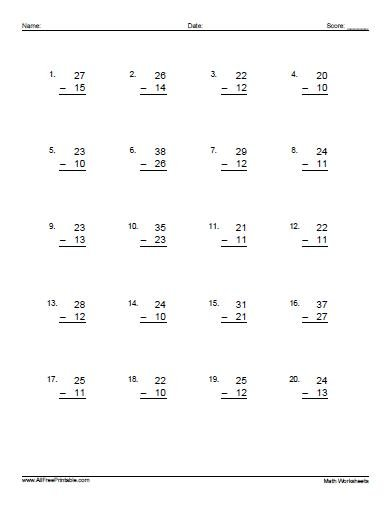# Subtraction Problems

Free Printable Subtraction Problems. Free Printable Subtraction Problems that teachers, educators or tutors can use for kids to help them improve subtraction skills. Save money printing the Subtraction Problems free using your home laser or inkjet printer, give to your kids and have fun learning math skills. We have 3 Subtraction Problems to choose from depending on the kid’s level of knowledge. Basic Subtraction for beginners at pre-k or kindergarten, Single Digit Subtraction and Two Digit Subtraction with regrouping and no regrouping problems. The Free Printable Subtraction Problems includes the answers on page 2 of PDF.